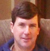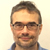# Carbon footprint of a HPWH| Posted in Mechanicals on

Following up on Dana’s blog post about the carbon footprint of mini-splits for space heating, I analyzed the carbon footprint of using a heat pump water heater. Of course, the analysis is full of assumptions. I present it here for people to comment on the reasonableness of my assumptions and methodology. If those withstand peer review, then the conclusions are useful.

To avoid having to analyze water consumption, I calculate the carbon emissions of putting 1,000,000 btus into the water. I assume that actions that reduce water consumption or standby losses will impact each option by the same proportion for each type.

Non-condensing natural gas storage water heater: Efficiency = 80%. NG CO2 = 117 lb CO2 / MMbtu (from Dana’s blog post). NGWH CO2 = (1 MMbtu)/(0.80)*(117 lb CO2/MMbtu) = 146 lb CO2.

Electric storage water heater: The CPP target for Michigan (from Dana’s article) is 1,169 lb CO2/MWh. EWH CO2 = (1 MMbtu)/(3.412 MMBtu/MWh)*(1169 lb CO2/MWh) = 343 lb CO2.

HPWH – winter: First, I need to calculate the carbon from the electric use. Assume COP = 2.5. Electric CO2 = (1 MMbtu)/(2.5)/(3.412 MMbtu/MWh)*(1169 lb/MWh) = 137 lb CO2. Then, I need to calculate the carbon of replacing the heat that the HPWH extracted from my conditioned space. Assume 95% efficient condensing natural gas furnace. Furnace CO2 = (1 MMbtu)*(1.5/2.5)/(0.95)*(117 lb CO2.MMbtu) = 74 lb CO2. Net HPWH CO2 = 137 + 74 = 211 lb CO2.

HPWH – summer: The carbon from the HPWH electric use is the same as winter. However, now it is offset by reduced use of the air conditioner. Assume 13 SEER air conditioner. AC CO2 credit = (1 MMbtu)*(1.5/2.5)/(13 MWh/MMbtu)*(1169 lb/MWh) = 54 lb CO2. Net HPWH CO2 = 137 – 54 = 83 lb CO2.

Conclusion: Pure electric is a bad choice. Relative to natural gas, a HPWH increases CO2 by 45% in the heating season and decreases CO2 by 43% in the cooling season. In Michigan, which has a longer heating season than cooling season, the NG WH is a little better. It isn’t a big enough difference for carbon footprint to drive a decision between NG and HPWH. It is close enough that modest changes in assumptions can change the result. If I change the HPWH COP to 3.0 instead of 2.5, then the HPWH increases CO2 by only 34% in winter and decreases it by 63% in summer. If my utility would satisfy the incremental electric load with 50% efficient combined cycle natural gas, then the CO2 from each MWh is (117 lb CO2/MMbtu NG)/(0.50)*(3.412 MMbtu/MWh) = 798 lb CO2/MWh. Under that assumption, at 2.5 COP, the HPWH is only 16% worse in winter and is 60% better in summer.## Join the leading community of building science experts

### Replies

1.Expert Member
| | #1

Another good application of crayon-on-napkin* math for estimating the size of the problem! Good on ya, Reid!

* Or was that calculation done with lipstick-on-mirror ? ;-)

• |
• |
• |
• |